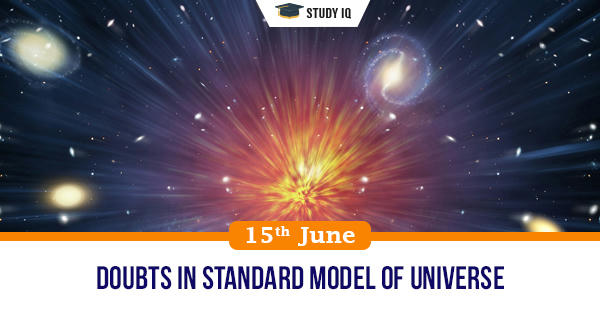# Current Affairs

## Filter By Tags

### Filter By Tags### Doubts in Standard model of universe

Date: 15 June 2020 Tags: Space

Issue

A new set of precision distance measurements made with an international collection of radio telescopes have greatly increased the likelihood that theorists need to revise the “standard model” that describes the fundamental nature of the Universe.

Background

The Standard Model is a set of mathematical formulae and measurements describing elementary particles and their interactions. It's similar to the way the Periodic Table of Elements describes atoms, categorizing them based on their characteristics, but instead the Standard Model categorizes the elementary particles - fermions and bosons.

Details

• The new distance measurements allowed astronomers to refine their calculation of the Hubble Constant, the expansion rate of the Universe, a value important for testing the theoretical model describing the composition and evolution of the Universe.

• The problem is that the new measurements highlights the discrepancy between previously measured values of the Hubble Constant and the value predicted by the model when applied to measurements of the cosmic microwave background made by the Planck satellite.

• There has been debate over whether this problem lies in the model itself or in the measurements used to test it. The work uses a distance measurement technique completely independent of all others, and it reinforces the disparity between measured and predicted values.

• Edwin Hubble first calculated the expansion rate of the universe (the Hubble Constant) in 1929 by measuring the distances to galaxies and their recession speeds.

• The more distant a galaxy is, the greater its recession speed from Earth. Today, the Hubble Constant remains a fundamental property of observational cosmology and a focus of many modern studies.

• Measuring recession speeds of galaxies is relatively straightforward. Determining cosmic distances, however, has been a difficult task for astronomers.

• A method of estimating the expansion rate involves observing distant quasars whose light is bent by the gravitational effect of a foreground galaxy into multiple images.

• When the quasar varies in brightness, the change appears in the different images at different times. Measuring this time difference, along with calculations of the geometry of the light-bending, yields an estimate of the expansion rate.

• Astronomers have various ways to adjust the model to resolve the discrepancy. Some of these include changing presumptions about the nature of dark energy, moving away from Einstein’s cosmological constant.

• Others look at fundamental changes in particle physics, such as changing the numbers or types of neutrinos or the possibilities of interactions among them.

• Testing the standard model of cosmology is a really challenging problem that requires the best-ever measurements of the Hubble Constant. The discrepancy between the predicted and measured values of the Hubble Constant points to one of the most fundamental problems in all of physics.